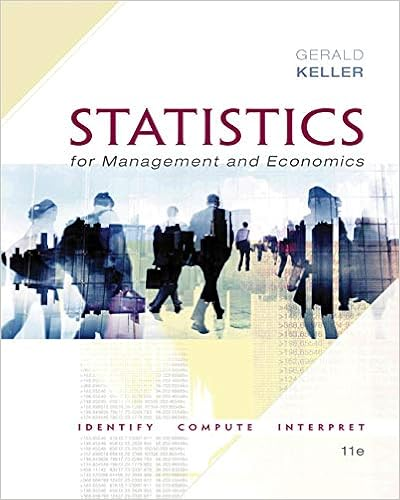# 9 nonlinear programming nlp ch 76 excel2010 textbook

• Notes
• yomen1
• 38
• 67% (3) 2 out of 3 people found this document helpful

This preview shows page 28 - 30 out of 38 pages.

##### We have textbook solutions for you!
The document you are viewing contains questions related to this textbook.The document you are viewing contains questions related to this textbook.
Chapter 22 / Exercise 22.8
Statistics for Management and Economics + XLSTAT Bind-in
KellerExpert Verified
9. Nonlinear programming (NLP), ch. 76 Excel2010 textbook: NLP arises when the objective or constraints are nonlinear; see, e.g., the EOQ model with the objective function V ( x ) = KD x + hx 2 . In Excel Solver, we pick the nonlinear method to solve it. 10. For Excel implementation, see Ch. 28-34 on Excel2010 textbook. 6.4 Exercise 1. Water Distribution: Suppose that each day, northern, central, and southern California each use 100 billion gallons of water. Also assume that northern California and central California each have available 120 billion gallons of water, whereas southern California has 40 billion gallons of water available. The cost of shipping 1 billion gallons of water between the three regions is as follows: Northern Central Southern Northern \$5,000 \$7,000 \$10,000 Central \$7,000 \$5,000 \$6,000 Southern \$10,000 \$6,000 \$5,000 You will not be able to meet all demand for water, so assume that each billion gallons of unmet demand incurs the following shortage costs: Northern Central Southern Shortage cost/billion \$6,000 \$5,500 \$9,000 gallons short How should California’s water be distributed to minimize the sum of shipping and shortage costs? HINTS: Take at your own risk. The total cost has two parts. The shipping cost is similar to the transporta- tion problem. To compute the shortage casts, we need to compute the shortage for each location. For example, let x ij be the shipment from origin i to destination j , where i, j ∈ { Norther = 1 , Central = 2 , Southern = 3 } . Let c ij be the unit shipping cost from i to j . Let x = [ x ij ] , c = [ c ij ] , where i, j = 1 , 2 , 3 . For region i = 1 , 2 , 3 , let s i , D i , and C i be its unit shortage cost, 28
##### We have textbook solutions for you!
The document you are viewing contains questions related to this textbook.The document you are viewing contains questions related to this textbook.
Chapter 22 / Exercise 22.8
Statistics for Management and Economics + XLSTAT Bind-in
KellerExpert Verified
water demand, and water capacity/supply. Hence, vectors s = ( s 1 , s 2 , s 3 ) = (6000 , 5500 , 9000) , D = (100 , 100 , 100) , and C = (120 , 120 , 40) . The shortage in region j is t j = D j ( x 1 j + x 2 j + x 3 j ) = D j 3 i =1 x ij , which costs s j · ( D j 3 i =1 x ij ) . Hence, the decision variable is x = [ x ij ] . The objective is to minimize V ( x ) = 3 i =1 3 j =1 c ij x ij | {z } total shipping cost + 3 j =1 s j · ( D j 3 i =1 x ij ) | {z } total shortage cost . The constraints are 3 j =1 x ij C i , i = 1 , 2 , 3 capacity constraints 3 i =1 x ij D j , j = 1 , 2 , 3 demand constraints In Excel, V = sumproduct ( c , x ) + sumproduct ( s , t ) where t = ( t 1 , t 2 , t 3 ) is the shortage vector. 2. Chip Manufacturing: At a chip manufacturing plant, four technicians (A, B, C, and D) produce three products (Products 1, 2, and 3). This month, the chip manufacturer can sell 80 units of Product 1, 50 units of Product 2, and at most 50 units of Product 3. Technician A can make only Products 1 and 3. Technician B can make only Products 1 and 2. Technician C can make only Product 3. Technician D can make only Product 2. For each unit produced, the products contribute the following profit: Product 1, \$6; Product 2, \$7; and Product 3, \$10. The time (in hours) each technician needs to manufacture a product is as follows: Product TechnicianA TechnicianB TechnicianC TechnicianD 1 2 2.5 Cannot do Cannot do 2 Cannot do 3 Cannot do 3.5 3 3 Cannot do 4 Cannot do Each technician can work up to 120 hours per month. How can the chip manufacturer maximize its monthly profit? Assume a fractional number of units can be produced.
•••